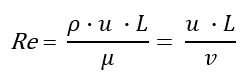# Reynolds Number Calculator

Easily calculate the Reynolds Number (Re) of a gas or liquid given its kinematic viscosity or dynamic viscosity and density, its velocity and characteristic length. Multiple metric and imperial measurement units are supported by the Reynolds Number calculator.

Share calculator:

Embed this tool:
get code

## Using the Reynolds Number calculator

With the help of this calculator you can compute the Reynolds Number of a liquid or gas. It supports a wide range of input and output measurement units (e.g. m2/s, Stokes and centiStokes for kinematic viscosity, imperial and metric/scientific units for density, velocity and length).

First, select whether you know the substance's kinematic viscosity or the dynamic viscosity and density and then enter the quantities you know. Then enter the velocity at which the substance is moving. Finally, enter the characteristic length, e.g. the inner diameter of a pipe, the diameter of a sphere moving in liquid, or the length of a plate over which the substance is flowing. Special consideration should be taken for fluids of variable density (e.g. compressible gases) and fluids of variable viscosity (non-Newtonian fluids).

The Reynolds Number calculator will then apply the relevant equation and produce the Reynolds Number (Re) as result.

## What is the Reynolds Number?

The Reynolds number (Re) is a dimensionless quantity for dynamic similarity and is calculated as the ratio of inertial forces to viscous forces of a flow of liquid. Knowing Re one can anticipate the transition from laminar to turbulent flow which is the main utility of a Reynolds Number calculator.

The number sees uses in fluid mechanics where it is used to predict flow patterns in different fluid flow scenarios. It can also be used to study and predict hot gases as in a flame in air. In practical applications the ability to predict when a turbulent flow will appear is important in designing piping systems, airplane wings, aerodynamic vehicles, including for scaling from study models to actual aircraft/vehicle. At larger scale it has uses in meteorology and climatology.

One interprets the Reynolds number as such: a low Reynolds number suggest the flow should be dominated by laminar (sheet-like) flow; at high Reynolds numbers one expects significant turbulence due to the differences in the fluid's speed and direction. Turbulence also leads to edgy currents in which different sub-flows intersect or even move counter to the overall flow direction. Edgy currents, in turn, use up energy and may produce cavitation.

While two flows with approximately equal Re can behave quite differently in practice due to their chaotic nature in which even small differences in shape and surface roughness can produce very different flows, the Reynolds number is still a useful and widely used guide to flow similarity.

## Reynolds Number Formula

Since the Reynolds equation describes the ratio between inertial forces and viscous forces acting on a flow it can be expressed both in terms of the kinematic viscosity or the dynamic viscosity and density of the substance:where ρ (Greek lower-case letter rho) is the density of the liquid (kg/m3), u is the velocity of the fluid relative to the object (m/s), μ (Greek lower-case letter mu) is the dynamic viscosity, v is the kinematic viscosity and finally L is the characteristic length (by convention - inner diameter of a pipe, diameter of a sphere in liquid, length of plate, etc.).

The formula was famously derived and demonstrated experimentally by Osborne Reynolds who studied the conditions in which the flow of fluid in pipes transitions from laminar flow to turbulent flow. The experiment was described in his 1883 paper: he used dyed water and studied its under different flow velocities when it mixed with clear water flow in a see-through pipe. This formula is the one we use to perform the math in this calculator.

### Dynamic viscosity units

Dynamic viscosity (or simply "viscosity") is the resistance to movement of one layer of a fluid over another for both shearing and non-shearing flows. The SI unit for dynamic viscosity is the Pascal-second, denoted "Pa s" or Pa·s to avoid confusion. One Pa·s equals 1 kg·m−1·s−1. The corresponding unit in the centimeter gram second system of units (cgs) is the poise (P) and 1P = g·m−1·s−1 = 0.1 Pa s . Often the centipoise (cP) is used as conveniently the viscosity of water at room temperature (20 °C) approximately equals one (1.0020 cP to be precise). Additionally, 1 cP = 1 mPa·s = 100 P. All of these are supported and automatically converted by our software.

### Kinematic viscosity units

The SI unit of kinematic viscosity is square meter per second (m2/s). A CGS unit named after Sir George Stokes is also used where one Stoke (St) = 0.0001 m2·s−1. Centistokes (cSt) is often used instead with 1 cSt = 1 mm2·s−1 = 10−6 m2·s−1. Conveniently, the kinematic viscosity of water at 20 °C is approximately equal to 1 cSt.

## Calculation Examples

Example 1: A sphere with a diameter of 2 cm is floating in a viscous liquid with dynamic viscosity of 5 kilogram-per-meter-second. The liquid's density is 900 kg/m3 and it is moving at a speed of 25 m/s. What is the Raynolds number for this flow?

To solve this we need to apply first variant of the Reynolds equation and replace the values: Re = 900 · 25 · 0.02 / 5 = 450 / 5 = 90.

Example 2: A plate has a length of 2 meters and a liquid with a kinematic viscosity of 250 Stokes is flowing with a speed of 10 m/s. What is the Reynolds number of this flow?

First, one must convert Stokes to m2/s by multiplying by 0.0001 and get 0.025 m2/s. We then substitute all values in the second equation and get Re = 10 · 2 / 0.025 = 20 / 0.025 = 800. If you use our Reynolds Number calculator, the conversion would be handled automatically.